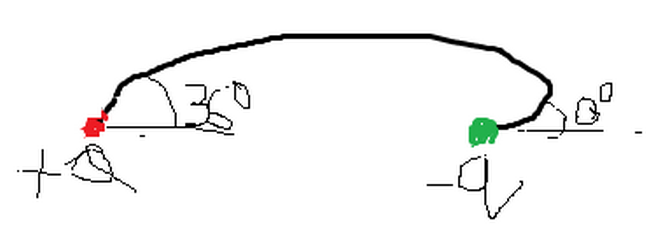# GuaSS law Might be Helpfull Here..!!Consider two charges $Q$ and $q$. An electrostatic electric field line exits the positive charge $Q$ at 36 degrees and enters the negative charge $q$ at 180 degrees, as shown in the figure above. What is the magnitude of ratio of charges $Q/q$?

Details and Assumptions

• Use $\sin(\pi/10)=0.309$
• The given figure is a rough idea for this problem (Plz feel it), and sorry for inconvenient figure.
• This is not original Question
×